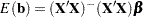Previous Page | Next Page

 The REG Procedure
 Models of Less Than Full Rank

If the model is not full rank, there are an infinite number of least squares solutions for the estimates. PROC REG chooses a nonzero solution for all variables that are linearly independent of previous variables and a zero solution for other variables. This solution corresponds to using a generalized inverse in the normal equations, and the expected values of the estimates are the Hermite normal form ofmultiplied by the true parameters:Degrees of freedom for the zeroed estimates are reported as zero. The hypotheses that are not testable havetests reported as missing. The message that the model is not full rank includes a display of the relations that exist in the matrix.

The following statements use the fitness data from Example 74.2. The variable Dif=RunPulseRestPulse is created. When this variable is included in the model along with RunPulse and RestPulse, there is a linear dependency (or exact collinearity) between the independent variables. Figure 74.48 shows how this problem is diagnosed.

```data fit2;
set fitness; Dif=RunPulse-RestPulse;
proc reg data=fit2;
model Oxygen=RunTime Age Weight RunPulse MaxPulse RestPulse Dif;
run;
```

Figure 74.48 Model That Is Not Full Rank: REG Procedure
The REG Procedure
Model: MODEL1
Dependent Variable: Oxygen

Analysis of Variance
Source DF Sum of
Squares
Mean
Square
F Value Pr > F
Model 6 722.54361 120.42393 22.43 <.0001
Error 24 128.83794 5.36825
Corrected Total 30 851.38154

 Root MSE R-Square 2.31695 0.8487 47.3758 0.8108 4.89057

Parameter Estimates
Variable DF Parameter
Estimate
Standard
Error
t Value Pr > |t|
Intercept 1 102.93448 12.40326 8.30 <.0001
RunTime 1 -2.62865 0.38456 -6.84 <.0001
Age 1 -0.22697 0.09984 -2.27 0.0322
Weight 1 -0.07418 0.05459 -1.36 0.1869
RunPulse B -0.36963 0.11985 -3.08 0.0051
MaxPulse 1 0.30322 0.13650 2.22 0.0360
RestPulse B -0.02153 0.06605 -0.33 0.7473
Dif 0 0 . . .

PROC REG produces a message informing you that the model is less than full rank. Parameters with DF=0 are not estimated, and parameters with DF=B are biased. In addition, the form of the linear dependency among the regressors is displayed.Previous Page | Next Page | Top of Page